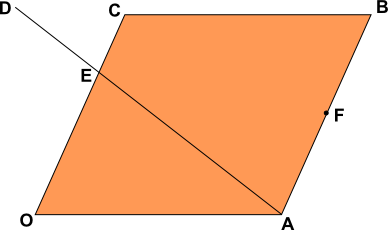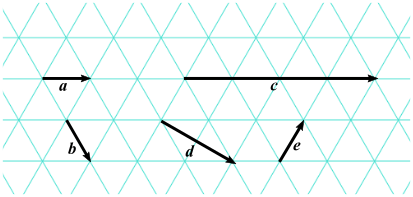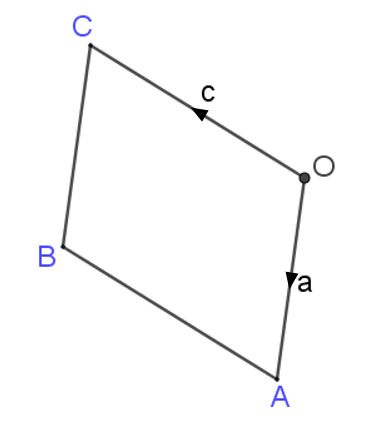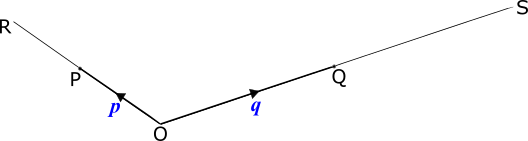# Exam-Style Questions on Vectors

## Problems on Vectors adapted from questions set in previous Mathematics exams.

### 1.

GCSE Higher

Calculate the vector $$3a - 2b$$ if the vectors $$a$$ and $$b$$ are:

$$a = \begin{pmatrix} -2 \\ -5 \\ \end{pmatrix}$$ $$b = \begin{pmatrix} -1 \\ -3 \\ \end{pmatrix}$$

### 2.

IGCSE Extended

OABC is a parallelogram with O as origin. The position vector of A is $$a$$ and the position vector of C is $$c$$.F is the mid-point of AB and the point E divides the line OC such that OE:EC = 2:1.

The point E also divides the line AD such that AE:ED = 3:2.

Find the following in terms of $$a$$ and $$c$$.

(a) $$\overrightarrow{OB}$$

(b) $$\overrightarrow{AC}$$

(c) $$\overrightarrow{AE}$$

(d) the position vector of F.

(e) $$\overrightarrow{AD}$$

(f) $$\overrightarrow{BD}$$

### 3.

GCSE Higher

(a) Shape $$A$$ is translated to shape $$B$$ using the vector $$\begin{pmatrix}m\\n\\ \end{pmatrix}$$. What are the values of $$m$$ and $$n$$?(b) Vectors $$a, b, c, d$$ and $$e$$ are drawn on an isometric grid. Write each of the vectors $$c, d$$ and $$e$$ in terms of $$a$$ and/or $$b$$.### 4.

GCSE Higher

ABCD is a quadrilateral. The points E, F, G and H are the midpoints of the sides of this quadrilateral.$$\vec{CH} = a, \vec{DG} = b \text{ and } \vec{DF} = c$$

Show that HG is parallel to EF.

### 5.

IGCSE ExtendedABCDOE is a regular hexagon with O as origin. The position vector of A is $$a$$ and the position vector of B is $$b$$.

Find the following in terms of $$a$$ and $$b$$.

(a) $$\overrightarrow{BA}$$

(b) $$\overrightarrow{OE}$$

(c) the position vector of C.

If the sides of the hexagon are each of length 10cm calculate:

(d) the size of angle $$BCD$$.

(e) the area of triangle $$BCD$$.

(f) the length of the line from B to D.

(g) the area of the hexagon.

### 6.

GCSE Higher

Consider a triangle ABC where M is the midpoint of AB and F is the point on BC where BF:FC = 3:4.(a) If $$\overrightarrow{AB}=b$$ and $$\overrightarrow{AC}=c$$ work out $$\overrightarrow{MF}$$ in terms of $$b$$ and $$c$$ giving your answer in its simplest form.

(b) Use your answer to part (a) to explain whether MF is parallel to AC or not.

### 7.

GCSE Higher

In the parallelogram OABC two of the sides can be represented by vectors $$a$$ and $$c$$.

$$\overrightarrow{OA} = a$$ and $$\overrightarrow{OC} = c$$$$X$$ is the midpoint of the line $$AC$$.

$$OCD$$ is a straight line such that $$OC:CD = k:1$$

Given that $$\overrightarrow{XD} = 3c - \frac12 a$$ find the value of $$k$$.

### 8.

IGCSE Extended

(a) If A is the point (3,5) write down the position vector of A.

(b) If B is the point (6,9) find $$\mid\overrightarrow{AB} \mid$$ the magnitude of $$\overrightarrow{AB}$$.

The following diagram is not to scale.$$O$$ is the origin, $$\overrightarrow{OP}=p$$ and = $$\overrightarrow{OQ}=q$$.

$$OP$$ is extended to $$R$$ so that $$OP=PR$$.

$$OQ$$ is extended to $$S$$ so that $$OQ=QS$$.

(c) Write down $$\overrightarrow{RQ}$$ in terms of $$p$$ and $$q$$.

(d) $$PS$$ and $$RQ$$ intersect at $$M$$ and $$RM=2 MQ$$.

Use vectors to find the ratio $$PM:PS$$, showing all your working.

### 9.

GCSE HigherIn the diagram above (not drawn to scale) $$X$$ is the point on $$AB$$ such that $$AX:XB = 9:4$$.

The position vector of $$A$$ is $$3a$$ and the position vector of $$B$$ is $$3b$$.

Find the value of $$k$$ if $$\vec{OX} = k(4a + 9b)$$ where $$k$$ is a scalar quantity.

### 10.

IB Standard

The line L is parallel to the vector $$\begin{pmatrix} 2 \\ 5 \\ \end{pmatrix}$$,

(a) Find the gradient of the line L .

The line L passes through the point (11, 3).

(b) Write down the equation of the line L in the form $$y=ax+b$$

(c) Find a vector equation for the line L.

### 11.

IB Standard

Consider two perpendicular vectors $$p$$ and $$q$$.

(a) Let $$r=p-q$$. Draw a diagram to show what this relationship might look like.

(b) If $$p=\begin{pmatrix} 4 \\ 1 \\ -3 \\ \end{pmatrix}$$ and $$q=\begin{pmatrix} 3 \\ n \\ -5 \\ \end{pmatrix}$$, where $$n\in \mathbb Z$$, find $$n$$.

### 12.

IB Standard

George and Hugo like to fly model airplanes. On one day George's plane takes off from level ground and shortly after that Hugo's plane takes off.

The position of George’s plane $$s$$ seconds after it takes off is given by $$\begin{pmatrix} 1 \\ 2 \\ 0 \\ \end{pmatrix} + s\begin{pmatrix} 5 \\ -2 \\ 6 \\ \end{pmatrix}$$ where the distances are in metres.

(a) Find the speed of George’s plane to the nearest integer.

(b) Find the height of George’s plane after four seconds.

The position of Hugo’s airplane $$t$$ seconds after it takes off is given by $$\begin{pmatrix} 4 \\ -4 \\ 0 \\ \end{pmatrix}+t\begin{pmatrix} 7 \\ -2 \\ 9 \\ \end{pmatrix}$$ where the distances are in metres.

(c) Show that the paths of the planes are not perpendicular.

The two airplanes collide at the point $$(46, -16, 54)$$.

(d) How long after George’s plane takes off does Hugo’s plane take off ?

### 13.

IB Standard

The line $$L_1$$ passes through the points A(3, 5, 1) and B(3, 6, 0).

(a) Show that $$AB=\begin{pmatrix} 0 \\ 1 \\ -1 \\ \end{pmatrix}$$

(b) Find a direction vector for $$L_1$$

(c) a vector equation for $$L_1$$

Another line $$L_2$$ has equation $$\begin{pmatrix} 8 \\ 3 \\ -2 \\ \end{pmatrix} + t \begin{pmatrix} -1 \\ 1 \\ 0 \\ \end{pmatrix}$$. The lines $$L_1$$ and $$L_2$$ intersect at the point P.

(d) Find the coordinates of P.

(e) Find a direction vector for $$L_2$$.

(f) Hence, find the angle between $$L_1$$ and $$L_2$$.

### 14.

IB Standard

Two points $$A$$ and $$B$$ have coordinates (1 , 3 , 6) and (8 , 7 , 10) respectively.

(a) Find $$\overrightarrow{AB}$$ in terms of the unit vectors $$i, j$$ and $$k$$.

(b) Find $$\mid\overrightarrow{AB} \mid$$

Let $$\overrightarrow{AC} = 5i + 2j - k$$

(c) Find the angle between $$AB$$ and $$AC$$.

(d) Find the area of triangle $$ABC$$.

(e) Hence or otherwise find the shortest distance from $$C$$ to the line through $$A$$ and $$B$$.

### 15.

A-Level

The points A and B have coordinates $$(3,-2,1)$$ and $$(4, 0, -1)$$ respectively.

The line $$l$$ has the following equation equation:

$$r= \begin{pmatrix} 3 \\ 2 \\ 0 \\ \end{pmatrix} + \lambda \begin{pmatrix} -1 \\ -2 \\ 0 \\ \end{pmatrix}$$

The point C lies on $$l$$ where $$\lambda = 3$$.

(a) Find the coordinates of C.

(b) Find the acute angle ABC, giving your answer to the nearest tenth of a degree.

(c) The point D lies on a line through A and B such that angle ADC is a right angle. Find the coordinates of D.

(d) The point E completes the parallelogram ACBE. Find the coordinates of E.

If you would like space on the right of the question to write out the solution try this Thinning Feature. It will collapse the text into the left half of your screen but large diagrams will remain unchanged.

The exam-style questions appearing on this site are based on those set in previous examinations (or sample assessment papers for future examinations) by the major examination boards. The wording, diagrams and figures used in these questions have been changed from the originals so that students can have fresh, relevant problem solving practice even if they have previously worked through the related exam paper.

The solutions to the questions on this website are only available to those who have a Transum Subscription.

Exam-Style Questions Main Page

Search for exam-style questions containing a particular word or phrase:

To search the entire Transum website use the search box in the grey area below.